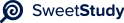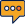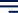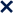•01 / 3100%1
Functions
îIn mathematics, a function is a relation between aîsetîof inputs and a set of permissible outputs
with the property that each input is related to exactly one output.
We define the following functions as:
f(x) = 2x+5, g(x) = x
2
-3, h(x) =(7-x)/3;
ï· In order to find out (f - h) (4), we need to find (f - h) and then evaluate the composite
function at x=4.
As f(x) = 2x+5 and h(x) =(7-x)/3;
(f- h)(x) is given by f(x) - h(x);
f(x) - h(x) = 2x+5 â(7-x)/3
= (3(2x+5) â (7 â x) ) / 3
=(6x + 15 â 7 + x ) / 3
= (7x + 8) / 3
We plug x = 4 in the above formulae to get the value of
(f - h)(4) = (7 * 4 + 8 ) / 3
= (28 + 8) / 3
= 36/3 = 12
Answer: (f - h) (4) =12.2
ï· We have to evaluate compositions (fog)(x) and (hog)(x);
We know that (f o g) (x) =f (g(x));
So, f (g(x)) =f(x
2
-3) = 2(x
2
â 3) + 5 = 2x
2
- 6 + 5 = 2x
2
- 1;
And similarly, (hog) (x) =h (g(x)) = h(x
2
â 3) = {7- (x
2
-3)}/3= {7- x
2
+ 3}/3 = (10-x
2
)/3.
ï· No we have to transform theîg(x)îfunction so that the graph is moved 6 units to the right and
7 units down;
The function of graph moved to right is given by g(x â c) (where c is the number of units
moved) here c = 6 so we get
g(x â 6) as the function representing graph moved 6 units right
The function of graph moved down is given by g(x) â c (where c is number of units
moved down) here c = 7 so we get
g(x) - 7 as the function representing graph moved 7 units down
so our final function becomes
g(x â 6) â 7
evaluating we get
g(x â 6) â 7 = (x â 6)
2
- 3 â 7
= x
2
â 12x + 36 â 10
= x
2
â 12x + 263
ï· îthe inverse functionsîîf
-1
î(x) and h
-1
(x) can be computed as follows,
the inverse function exists only if the function is bijective. The input and output variable are
swapped.
f(x) = 2x+5
replacing x and f(x) and then solving for f(x) to get the inverse
x = 2*f(x) + 5
2*f(x) = x â 5
f(x) = (x â 5) / 2
so inverse is f
-1
(x) = (x â 5) / 2
h(x)=(7 - x)/3
replacing x and f(x) and then solving for f(x) to get the inverse
x={7-h(x)}/3
7 â h(x) = 3x
h(x) = 7 â 3x
so inverse is h
-1
(x) = 7 â 3x
References:
1> Elementary and Intermediate Algebra